“Let our rigorous testing and reviews be your guidelines to A/V equipment – not marketing slogans”

# Lowering Mechanical Noise Floor in Speakers - page 2

By

The Mathematics

The classic equation for determining the resonance frequencies of a uniform, isotropic, rectangular panel, with boundary conditions set as all edges, simply supported (ss) is:

and for the same panel with boundary conditions set as all edges clamped (c), the fundamental resonance frequency can be found with:

Where: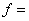Resonance frequency, Hz

D =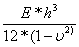, flexural rigidity or bending stiffness of plate

m, n = mode numbers, integers

ρ = panel mass per unit area

E = Young's modulus, modulus of elasticity

h = panel thickness

a = panel length

b = panel width

ν = Poisson's ratio (ratio of orthogonal straining)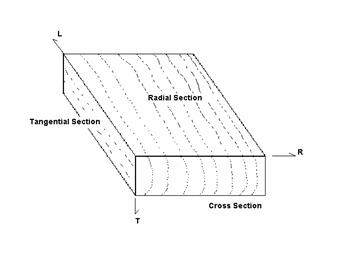A few caveats are in order where it comes to these equations. First, note the phrase "isotropic". Isotropic means a material that exhibits mechanical properties (such as Young's modulus) that are independent of which direction the measurements are taken from which the mechanical properties are calculated. A good example of an isotropic material would be MDF or, as mentioned previously, a polymer composite material.

A material such as plywood would not be considered isotropic, but rather orthotropic, which means it's measured mechanical properties are dependent on the direction (laterally, transversely or radially) being considered.

A second caveat is in order: when you start cutting openings in the panel, the formulae are no longer applicable and measured versus calculated resonance values will differ substantially. This doesn't mean the formulae aren't useful in speaker building, they are. But I would use them only as a starting point for working up a rough estimate of panel resonance frequency for those panels that don't have openings cut into them and only if I were using an isotropic material such as MDF or a polymer composite.

As mentioned earlier, the contribution to total system acoustic output made by the panels making up a loudspeaker cabinet does not arise solely from the mechanical vibrations set up in each panel by the motion of the driver's frame and other adjacent panels. Another factor, the outward transmission by the outer side of a panel due to fluctuating pressure incident to the internal side of a cabinet panel needs to be considered. For following analysis, it is again assumed the panels behave as plates, clamped at each edge and under uniform load by the driver diaphragm-induced acoustic pressure fluctuations.

In any case, the internal pressure fluctuations found within a loudspeaker cabinet under normal operating conditions aren't very large to begin with and further decrease as frequency increases. The internal box pressure created by an operational driver bolted in to one panel of the cabinet can be found, to a good first approximation, by:

Where:

Pb = Rms box pressure, Pascals

Pa = radiated acoustic power, Watts

c = velocity of sound in air, ~345 m/s

ρ o = density of air, 1.18 kg/m^3

Vb = net internal box volume, Liters

f = frequency, Hz

Running a series of simulations, holding Pa constant at .1W and varying Vb, using the above equation well illustrates this:

One commonly seen mathematical method used for expressing the degree to which a panel can reduce the outward expression of these internal pressure fluctuations is by transmission loss.

Transmission loss, in dB, is expressed mathematically by:

Where τ , the transmission loss coefficient, is expressed by: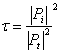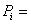incident pressure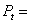transmitted pressure

Factoring in panel mass and frequency, the TL equation then becomes:

Where:

m = panel surface density, ( ρ * h)

ρ = material density

h = panel thickness

ω = radian frequency

z = panel material impedance

Taking into account m, and solving for B, the bulk modulus or effective stiffness of the panel is found by:

And from the results of the preceding equation we can now calculate the panel's critical frequency:

Finally, we can finally calculate, the transmission loss slope below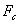/2:

And the slope above:

Confused about what AV Gear to buy or how to set it up? Join our Exclusive Audioholics E-Book Membership Program!